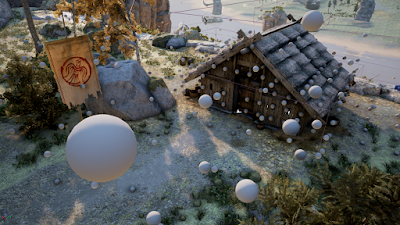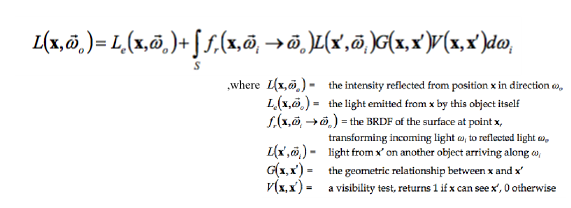## Wednesday, 15 November 2017

### Spherical Harmonics in Graphics: A Brief OverviewVisual representation of Spherical Harmonics

Global Illumination is a very popular and demanding topic in computer graphics. In particular, real-time graphics developers have been struggling with it for over 10 years now, still optimizing and implementing new techniques for modelling indirect lighting. Today I want to take all the articles, blogs and papers together and review one very efficient method of storing light information - Spherical Harmonics, or more precisely, the method that actually encodes the light data. For example, there can be a common situation when light probes should gather data and SH will help to compress it (i.e. Unity is working with the same approach).Example of a scene with light probes

Spherical Harmonics are quite difficult to understand without proper math background, so that is why I will try to describe them as easy as possible here. First of all, you may ask why the heck do we need these harmonics at all? Let's take a look at the main rendering equation:Arrghh... You said "easy"!!!

Don't worry, it can be simplified for our real-time purposes to something like this:Lambert diffuse without shadows

So we need to solve this integral by finding L(x,w) and max(N*w, 0). That's where exactly SH are going to help us. They can be used as projecting inputs for our 2 functions from the integral above. But how? Let's find out! This intensity function can be estimated with Monte Carlo Integration. I'm not going to dive into the details (its a topic of probability), but the overall idea is that we can approximate our integral by calculating a dot product of 2 SH coefficients. Actually, this property of SH  makes them so efficient!

Now let's quickly talk about Spherical Harmonics themselves to get a better understanding of the process. They come from solving Laplace's equation and describe a set of orthogonal basis functions in spherical coordinates. Basis simply means that these functions can be scaled and combined together to approximate an original function (yeah, like Fourier). Orthogonal (polynomials) have a unique feature: when we integrate the product of any 2 similar of them we get a constant and when we integrate 2 different - we get 0. There are several polynomials of this type, but we are going to work with Associated Legendre Polynomials (just read about them on the Internet). So in general SH then look like this (where l is an integer (band index) that is >=0 and m is 0<=m<=l):

In addition, I want to mention that in real-time graphics 2 and 3 bands are enough, as they already give us desirable results.

That is it! Finally, we can compute our approximated integral using the equation above. Actually, there is a lot more to mention about SH, for example, rotational invariance, zonal harmonics or the fact that this method is only good for low-frequency compression. This time I've only tried to make an overview of Spherical Harmonics! So if you are interested more, you should definitely read the original posts and publications down below. I will also attach the link to a series about another similar, but newer, approach - Spherical Gaussians used in The Order: 1886 by Ready at Dawn. Now check out the shader by Morgan McGuire: